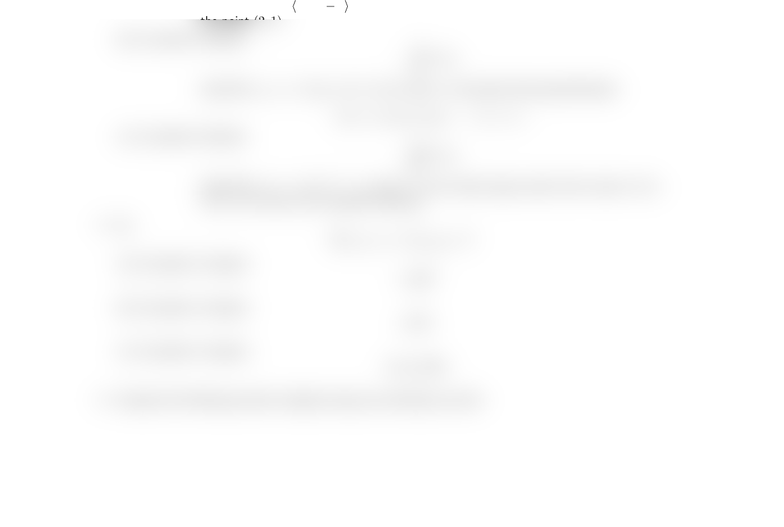# Math 2153 Final Exam

57 views2 pages
School
Ohio State University
Department
Mathematics
Course
MATH 2153
ProfessorPractice Final Exam – Math 2153
1. Decide if the following statements are TRUE or FALSE and circle your answer. You do NOT need to justify
(a) (1 point) If line integrals in the continuous vector ﬁeld F(x, y)are path independent then Fis a
conservative vector ﬁeld.
(b) (1 point) If Fis a conservative vector ﬁeld then line integrals in the continuous vector ﬁeld F(x, y)
are path independent.
(c) (1 point) If F(x, y)has continuous ﬁrst partial derivatives on the connected, simply connected
region Rand F(x, y)is irrotational then Fis conservative.
(d) (1 point) If F(x, y)has continuous ﬁrst partial derivatives on the connected, simply connected
region Rand F(x, y)is source-free then Fis conservative.
2. Give examples of the following. Be as explicit as possible. You do NOT need to justify your answers.
(a) (2 points) Give an example of a scalar function f(x, y)whose implicit domain is connected but
not simply connected.
(b) (2 points) Give an example of a non-constant conservative vector ﬁeld F(x, y, z)with domain R3.
(c) (2 points) Give an example of parametrized path in R2which is not a simple path.
(d) (2 points) Give an example of a non-constant source-free vector ﬁeld F(x, y, z)with domain R3.
3. Compute the following line integrals using any technique you like:
(a) (5 points) Evaluate ZC
F·dr
where F(x, y) = hxy, x yiand Cis the straight line segment from the point (0,0) to
the point (2,1).
(b) (2 points) Evaluate ZC
F·dr
where F(x, y, z) = h6xyz, 3x2z, 3x2yiand Cis the path with parametrization
r(t) = ht, sin t, t sin ti0tπ
(c) (2 points) Evaluate IC
F·dr
where F(x, y) = hxy2, x2yiand Cis the closed square path with corners (0,0),
(0,2),(2,2) and (2,0) oriented clockwise.
4. Let
F(x, y, z) = hx2y, xyz, z2i
(a) (5 points) Compute
curl F
(b) (5 points) Compute
div F
(c) (5 points) Compute
div(curl F)
5. Compute the following surface integrals using any technique you like:
Unlock document

This preview shows half of the first page of the document.
Unlock all 2 pages and 3 million more documents.